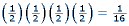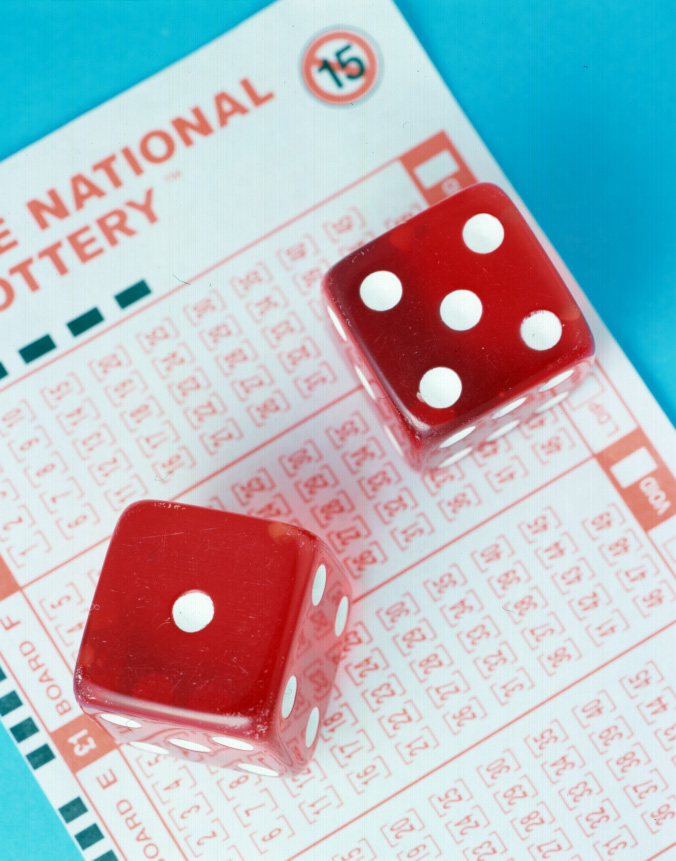People often misunderstand the notion of independent events.  This is a probability term meaning that past events have no influence on future outcomes.  For example, when flipping a coin four consecutive times, the probability of getting four heads is:This is because the probability of flipping a head if you flip a coin once is ½.  Flipping a coin is an example of an independent event.  When flipping a coin, the probability of getting a head does not change no matter how many times you flip the coin.  When the coin is flipped and the first three flips are heads, the fourth flip still has the probability of ½   However, many people misunderstand that the first three flips somehow influence the fourth flip, but they do not.  The probability is still the same, as if the first three flips had never occurred.

This is a common misunderstanding when people are picking numbers for a lottery.  Picking the same numbers every time does not guarantee that they will be picked eventually.  Also, if a person picked 36 as one of their numbers and one of the drawn numbers was 35, it does not really imply that the person was close to winning.  Although 35 and 36 are only one number apart, it does not mean that after 35 was picked, 36 would be the next number.  In fact, the person is no closer than if 3 or 97 were picked.  People often think this way, but it is just a misconception of independent events.Image reproduced with permission of DHD Multimedia Gallery

Gambling card games are not necessarily independent events.  If the cards are not replaced into the deck, then probabilities change depending on which cards have been dealt.  For example, the probability of being dealt an ace from a standard deck of 52 cards is 4/52  or 1/13.  However, if the first person is dealt an ace, the probability that the second person will also be dealt an ace is now 3/51, if the first ace is not replaced into the deck.  This applies to all card games, particularly Poker.